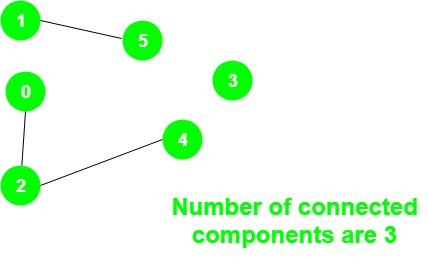# Program to count Number of connected components in an undirected graph

Given an undirected graph g, the task is to print the number of connected components in the graph.

Examples:

Input:Output: 3
There are three connected components:
1 – 5, 0 – 2 – 4 and 3

## Recommended: Please try your approach on {IDE} first, before moving on to the solution.

Approach: The idea is to use a variable count to store the number of connected components and do the following steps:

1. Initialize all vertices as unvisited.
2. For all the vertices check if a vertex has not been visited, then perform DFS on that vertex and increment the variable count by 1.

Below is the implementation of the above approach:

 `// C++ implementation of the approach ` `#include ` `using` `namespace` `std; ` ` `  `// Graph class represents a undirected graph ` `// using adjacency list representation ` `class` `Graph { ` `    ``// No. of vertices ` `    ``int` `V; ` ` `  `    ``// Pointer to an array containing adjacency lists ` `    ``list<``int``>* adj; ` ` `  `    ``// A function used by DFS ` `    ``void` `DFSUtil(``int` `v, ``bool` `visited[]); ` ` `  `public``: ` `    ``// Constructor ` `    ``Graph(``int` `V); ` ` `  `    ``void` `addEdge(``int` `v, ``int` `w); ` `    ``int` `NumberOfconnectedComponents(); ` `}; ` ` `  `// Function to return the number of ` `// connected components in an undirected graph ` `int` `Graph::NumberOfconnectedComponents() ` `{ ` ` `  `    ``// Mark all the vertices as not visited ` `    ``bool``* visited = ``new` `bool``[V]; ` ` `  `    ``// To store the number of connected components ` `    ``int` `count = 0; ` `    ``for` `(``int` `v = 0; v < V; v++) ` `        ``visited[v] = ``false``; ` ` `  `    ``for` `(``int` `v = 0; v < V; v++) { ` `        ``if` `(visited[v] == ``false``) { ` `            ``DFSUtil(v, visited); ` `            ``count += 1; ` `        ``} ` `    ``} ` ` `  `    ``return` `count; ` `} ` ` `  `void` `Graph::DFSUtil(``int` `v, ``bool` `visited[]) ` `{ ` ` `  `    ``// Mark the current node as visited ` `    ``visited[v] = ``true``; ` ` `  `    ``// Recur for all the vertices ` `    ``// adjacent to this vertex ` `    ``list<``int``>::iterator i; ` ` `  `    ``for` `(i = adj[v].begin(); i != adj[v].end(); ++i) ` `        ``if` `(!visited[*i]) ` `            ``DFSUtil(*i, visited); ` `} ` ` `  `Graph::Graph(``int` `V) ` `{ ` `    ``this``->V = V; ` `    ``adj = ``new` `list<``int``>[V]; ` `} ` ` `  `// Add an undirected edge ` `void` `Graph::addEdge(``int` `v, ``int` `w) ` `{ ` `    ``adj[v].push_back(w); ` `    ``adj[w].push_back(v); ` `} ` ` `  `// Driver code ` `int` `main() ` `{ ` `    ``Graph g(5); ` `    ``g.addEdge(1, 0); ` `    ``g.addEdge(2, 3); ` `    ``g.addEdge(3, 4); ` ` `  `    ``cout << g.NumberOfconnectedComponents(); ` ` `  `    ``return` `0; ` `} `

Output:

```2
```

GeeksforGeeks has prepared a complete interview preparation course with premium videos, theory, practice problems, TA support and many more features. Please refer Placement 100 for details

My Personal Notes arrow_drop_upCheck out this Author's contributed articles.

If you like GeeksforGeeks and would like to contribute, you can also write an article using contribute.geeksforgeeks.org or mail your article to contribute@geeksforgeeks.org. See your article appearing on the GeeksforGeeks main page and help other Geeks.

Please Improve this article if you find anything incorrect by clicking on the "Improve Article" button below.